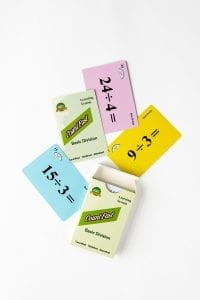# Concept of +- (1-12) Home Activities

####OVERVIEW & PURPOSE

Week 6 of the 1st Grade CountFast program focuses on addition and subtraction using the digits 6 through 12.  This module includes lessons on how to play an addition and subtraction game using the card deck.  Spend 15 minutes each day on one of the activities listed in this module.  Card decks should go home with students each day for additional practice with a parent at home. Each week, a new deck is introduced and the previous deck is for the student to keep at home for continued practice.

#### EDUCATION STANDARDS

1. NCTM Standard: develop and use strategies for whole-number computations, with a focus on addition and subtraction
2. NCTM Standard: understand the effects of adding and subtracting whole numbers
3. MATH.CONTENT.K.OA.A.1:  Represent addition and subtraction with objects, fingers, mental images, drawings1, sounds (e.g., claps), acting out situations, verbal explanations, expressions, or equations.
4. MATH.CONTENT.1.OA.D.7:  Understand the meaning of the equal sign, and determine if equations involving addition and subtraction are true or false.

#### OBJECTIVES

1. Sort the numbers 6 through 24 in least-to-greatest and greatest-to-least form.
2. Create accurate addition and subtraction equations using the numbers 6-24.
3. Understand and use the symbols for equal, add (plus), and subtract (minus).
DAY 1:Using the yellow cards in this week’s deck, continue to have your child practice putting the numbers in order from least to greatest and from greatest to least.  With the yellow cards laid out in number order, ask your child questions about “how many more” or “how many fewer” some of the numbers are from each other.  This is a review of the concepts learned in the 2nd week of the program, but now we are using larger numbers.DAY 2 AND 3:

Using the blue cards of the deck, ask your child to build simple addition equations and solve them.  (Ex:  6 + 7 =13, 8 + 9 = 17, etc.)  Call out the problems and let your child build/solve.  After some practice, challenge your child to create 7 different addition equations (problems) for you to see.  Use the ‘Addition Time Record’ card to keep track of how long it takes to create and solve the 7 equations.  Use this card daily to see if your child can build and solve the equations faster and faster each time.

DAY 4 AND 5:

Using the pink cards of the deck, ask your child to build simple subtraction equations and solve them.  (Ex:  20 – 8 = 12, 24 – 9 = 15, etc.)  Call out the problems and let your child build/solve.  After some practice, challenge your child to create 7 different subtraction equations (problems) for you to see.  Use the ‘Subtraction Time Record’ card to keep track of how long it takes your child to create and solve the 7 equations.  Use this card daily to see if your child can build and solve the equations faster and faster each time.

**Note**

In class this week, we are discussing how we can reverse the numbers of an addition problem and get the same answer.  This is called the Commutative Property of Addition (2 + 3 equals the same as 3 + 2).  We are also learning that this concept does NOT work for subtraction.  Our subtraction problems should begin with a larger number than the number we are taking away.  With addition, we are ‘counting up’.  With subtraction, we are finding the ‘difference’ between the two numbers.

While we are learning and reviewing the concepts of what addition and subtraction mean, the main focus of these activities is to increase mental calculation accuracy and speed.  Each time you do an activity with your child, encourage him/her to complete the activity a little faster than the previous time.  Use the time record cards to show your child how much faster he/she is answering each day.  At the end of the week, plan a fun way to celebrate that improvement together!

Stay SharpFree flash card decks to keep kids entertained and sharp!

To help you keep your kids math sharp during this crisis, we're giving you 3 free CountFast flash card decks for just \$6 in shipping.  Just have them take the quick quiz for their grade at the link below and you'll get a discount code to use on any three decks.